•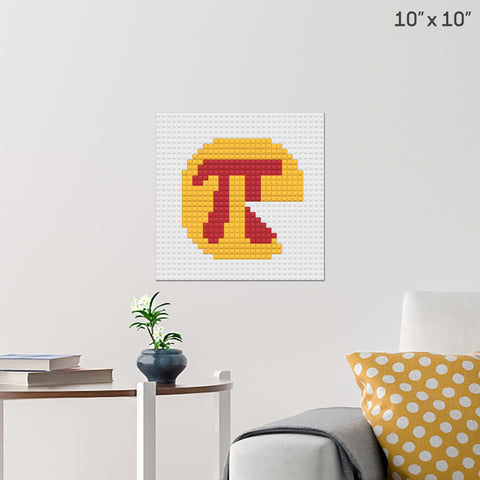### Pi Day Brick Poster

Pi Day is an annual celebration of the mathematical constant π. See more

Posted by

•### Square Root Brick Poster

In mathematics, a square root of a number a is a number y such that y2 = a; in other words, a number y whose square (the result of multiplying the ... See more

Posted by

•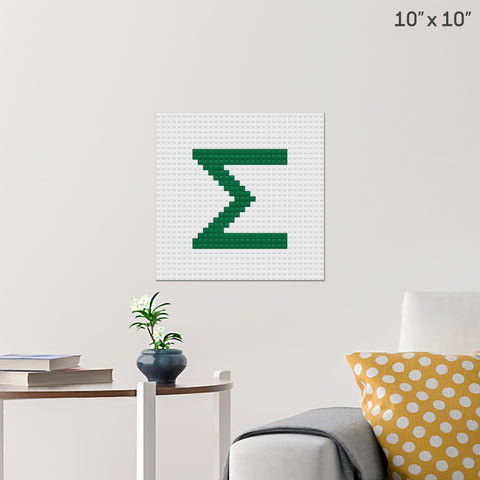### Sigma Brick Poster

In addition to being the 18th letter of the Greek alphabet, sigma also means 'sum' and 'deviation' in themathematics world. See more

Posted by

•### Plus Sign Brick Poster

The plus sign is written as a cross of horizontal and vertical lines: + The plus sign indicates addition operation of 2 numbers or expressions. See more

Posted by

•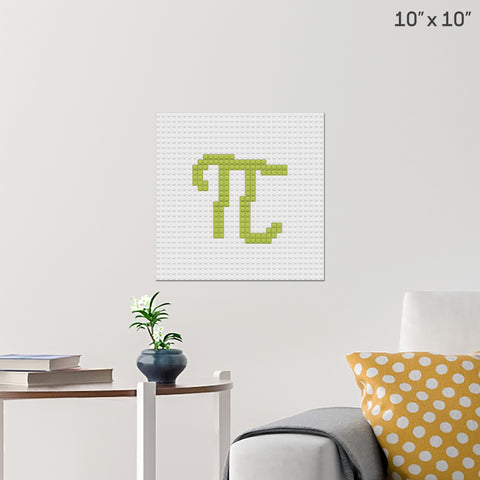### Pi Brick Poster

It is approximately equal to 3.14159. It has been represented by the Greek letter "π" since the mid-18th century, though it is also sometimes spell... See more

Posted by

•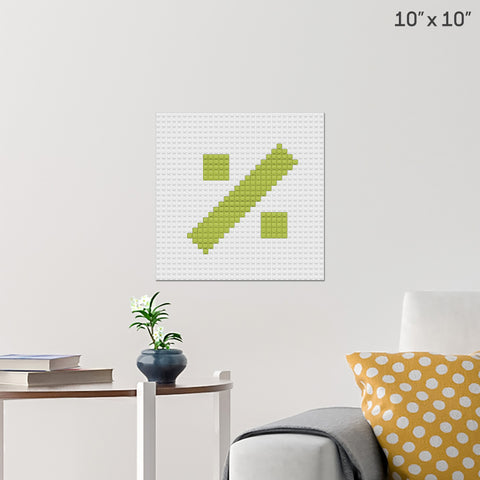### Percent Sign Brick Poster

The percent (per cent) sign (%) is the symbol used to indicate a percentage, a number or ratio as a fraction of 100. See more

Posted by

•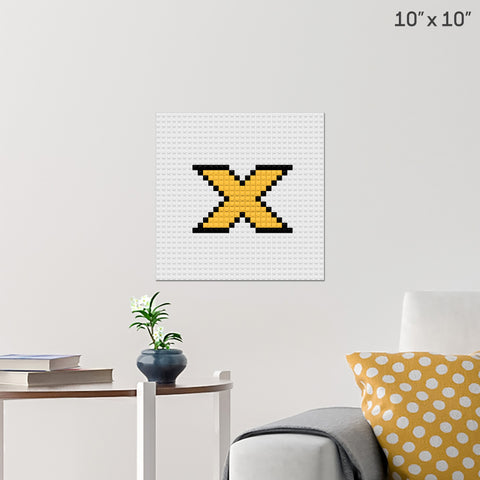### Multiplication Sign Brick Poster

The multiplication sign, also known as the times sign or the dimension sign is the symbol ×. See more

Posted by

•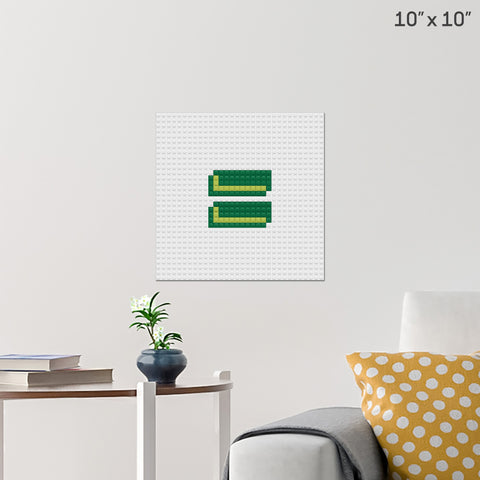### Equal Sign Brick Poster

The equals sign or equality sign (=) is a mathematicalsymbol used to indicate equality. See more

Posted by

•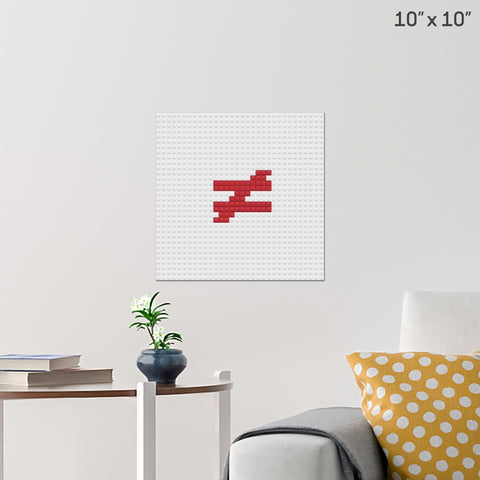### Inequality Sign Brick Poster

In mathematics, an inequality is a relation that holds between two values when they are different. The notation a ≠ b means that a is not equal to b. See more

Posted by

•### Minus Sign Brick Poster

A minus sign is put between two numbers in order to show that the second numberis being subtracted from the first one. See more

Posted by

•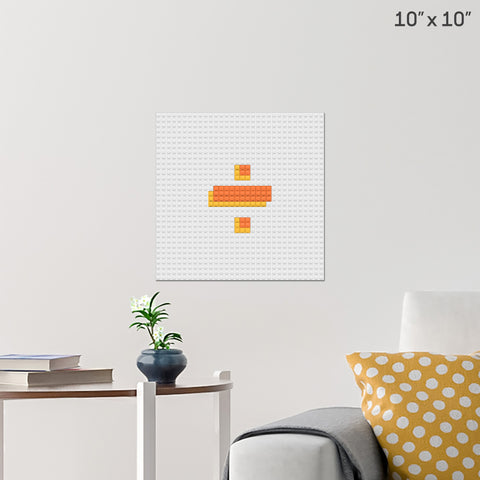### Division Sign Brick Poster

An obelus is a symbol consisting of a short horizontal line with a dot above and below. See more

Posted by